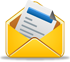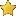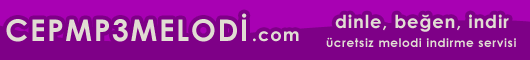Hoşgeldiniz, bugün 24 February 2020
aktif üye 8,903
SEN DE YAZ
HazırKod ziyaretçilerinin katkıları bizim için çok önemli!Sizde hazırkod üyesi olun, ekleyeceğiniz kodlar yapacağınız yorumlarla hazirkod.com'a katkıda bulunun.
ETİKETLER

# Programalama > C#

Etiketler: klasik, duvar, saatiOrt. 5
Puan ver:
```using System;
using System.Windows.Forms;
using System.Drawing;

class MyForm : Form
{
MyForm ()
{
Text = "Clock";
SetStyle (ControlStyles.ResizeRedraw, true);
}

protected override void OnPaint (PaintEventArgs e)
{
SolidBrush red = new SolidBrush (Color.Red);
SolidBrush white = new SolidBrush (Color.White);
SolidBrush blue = new SolidBrush (Color.Blue);

// Initialize the transformation matrix
InitializeTransform (e.Graphics);

// Draw squares denoting hours on the clock face
for (int i=0; i<12; i++) {
e.Graphics.RotateTransform (30.0f);
e.Graphics.FillRectangle (white, 85, -5, 10, 10);
}

// Get the current time
DateTime now = DateTime.Now;
int minute = now.Minute;
int hour = now.Hour % 12;

// Reinitialize the transformation matrix
InitializeTransform (e.Graphics);

// Draw the hour hand
e.Graphics.RotateTransform ((hour * 30) + (minute / 2));
DrawHand (e.Graphics, blue, 40);

// Reinitialize the transformation matrix
InitializeTransform (e.Graphics);

// Draw the minute hand
e.Graphics.RotateTransform (minute * 6);
DrawHand (e.Graphics, red, 80);

// Dispose of the brushes
red.Dispose ();
white.Dispose ();
blue.Dispose ();
}

void DrawHand (Graphics g, Brush brush, int length)
{
// Draw a hand that points straight up, and let
// RotateTransform put it in the proper orientation
Point[] points = new Point;
points.X = 0;
points.Y = -length;
points.X = -12;
points.Y = 0;
points.X = 0;
points.Y = 12;
points.X = 12;
points.Y = 0;

g.FillPolygon (brush, points);
}

void InitializeTransform (Graphics g)
{
// Apply transforms that move the origin to the center
// of the form and scale all output to fit the form's width
// or height
g.ResetTransform ();
g.TranslateTransform (ClientSize.Width / 2,
ClientSize.Height / 2);
float scale = System.Math.Min (ClientSize.Width,
ClientSize.Height) / 200.0f;
g.ScaleTransform (scale, scale);
}

static void Main ()
{
Application.Run (new MyForm ());
}
}```Yorumlar                 Yorum Yaz
Bu hazır kod'a ilk yorumu siz yapın!
KATEGORİLER
ASP - 240
ASP.NET - 24
C# - 75
C++ - 174
CGI - 8
DELPHI - 247
FLASH - 49
HTML - 536
PASCAL - 246
PERL - 11
PHP - 160
WML - 9
XML - 2